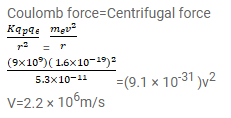# In the Bohr model of hydrogen atom,

Question:

In the Bohr model of hydrogen atom, the electron is treated as a particle going in a circle with the centre at the proton. The proton itself is assumed to be fixed in an inertial frame. The centripetal force is provided by the Coulomb attraction. In the ground state, the electron goes round the proton in a circle of radius $5.3 \times 10^{-11} \mathrm{~m}$. Find the speed of the electron in the ground state. Mass of the electron $=9.1 \times 10^{-31} \mathrm{~kg}$ and charge of the electron $=1.6 \times 10^{-19} \mathrm{C}$.

Solution: